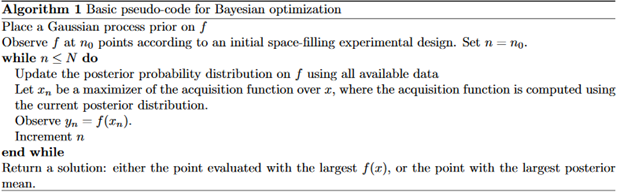The term bayesian comes from thomas bayes, a well known statistician from the 18th century, he is famous for establishing the bayes rule.

The term bayesian comes from thomas bayes, a well known statistician from the 18th century, he is famous for establishing the bayes rule. Bayesian optimization is a technique of optimization method focused on bringing the might of probability whenever the deterministic approach can’t solve any engineering problem at hand, it is applied to a plethora of domains and applications. In our context it is applied to the optimization of hyperparameters by approaching the latter as a prior belief and expect them to behave in a certain way, after that a search in the domain of that prior is executed and some measures are taken to update the prior. In the literature the set of hyperparameters to be tuned are considered to be linked to an unknown function or a black box since there is an explanation of how they affect the model or even how they interact with each other.

This process gives us two ways of harvesting the hyperparameters: exploration and exploitation, the exploration roams the so called domain space in search for most relevant corners of the space and exploitation which is the process of scanning for the most promising values in the spotted areas of search.

Due to its nature, the BO is considered to be an informed method of optimization because it builds on the search space already explored and try to optimize it and focus on the promising areas and ignore the less significant areas.

The first paper to introduce BO for hyperparameter optimization is “Practical Bayesian Optimization of Machine Learning Algorithms” by Snoek et al,. It showed that bayesian optimization could outperform expert like skills at finding the optimal set of hyperparameters.

Bayesian optimization consists of two main components:

•  A Bayesian statistical model for modeling the objective function, in the
literature it is called a probabilistic surrogate model.
• An acquisition function for deciding where to sample next (Feurer, 2018).

After evaluating the objective according to an initial space filling experimental design, often consisting of points chosen uniformly at random, they are used iteratively to allocate the remainder of a budget of N function evaluations, as shown in this Algorithm(Frazier, 2018):Bayesian optimization in reality:

BO can be broken down into these steps:

1. Build a surrogate probability model of the objective function
2. Find the hyperparameters that perform best on the surrogate
3. Apply these hyperparameters to the true objective function
4. Update the surrogate model incorporating the new results
5. Repeat steps 2–4 until max iterations or time is reached

So we have here 3 fancy words the surrogate, the objective function and another word mentioned above in this article, the acquisition function. The surrogate is a form of a probabilistic model mapping hyperparameters to a probability of a score on the objective function:

P (score | hyperparameters)

It is much easier and computationally cheaper to optimize the surrogate than the objective function because the concept is to limit evaluations of the objective function by spending more time choosing the next values to try. There are 3 common types of the surrogate models: Gaussian Processes, Random Forest Regressions, and Tree Parzen Estimators (TPE).

The objective function f(x) is what we are trying to optimize, this function doesn’t have an analytical expression nor do we know its derivatives and is non convex which means it has multiple local minimas. It can take the form of the validation loss, accuracy.

The acquisition or selection function or utility function can be:

• The upper confidence bound UCB.
• The probability of improvement PI..
• The expected improvement EI, which is the popular one and the mostly used across research articles.

Sources:

A Tutorial on Bayesian Optimization; Frazier;  2018

Practical Bayesian Optimization of Machine Learning Algorithms; Snoek et al ; 2012

A Conceptual Explanation of Bayesian Hyperparameter Optimization for Machine Learning; “https://towardsdatascience.com/a-conceptual-explanation-of-bayesian-model-based-hyperparameter-optimization-for-machine-learning-b8172278050f”; 2018

Using Bayesian Optimization to reduce the time spent on hyperparameter tuning; Kraus, Mike; “https://medium.com/vantageai/bringing-back-the-time-spent-on-hyperparameter-tuning-with-bayesian-optimisation-2e21a3198afb”; 2019

author : Ettaik Noureddine  PhD Condidate At FSBM